# Tutorial: Error Estimation - Part 2 (Autocorrelation Analysis)¶

## Data generation¶

This first code cell will provide us with the same two data sets as in the previous part of this tutorial. We will use them to get familiar with the autocorrelation analysis method of error estimation.

In :
import numpy as np
%matplotlib inline
import matplotlib.pyplot as plt

In :
plt.rcParams.update({'font.size': 18})
import sys
import logging
logging.basicConfig(level=logging.INFO, stream=sys.stdout)

np.random.seed(43)

def ar_1_process(n_samples, c, phi, eps):
'''
Generate a correlated random sequence with the AR(1) process.

Parameters
----------
n_samples: :obj:int
Sample size.
c: :obj:float
Constant term.
phi: :obj:float
Correlation magnitude.
eps: :obj:float
Shock magnitude.
'''
ys = np.zeros(n_samples)
if abs(phi) >= 1:
raise ValueError("abs(phi) must be smaller than 1.")
# draw initial value from normal distribution with known mean and variance
ys = np.random.normal(loc=c / (1 - phi), scale=np.sqrt(eps**2 / (1 - phi**2)))
for i in range(1, n_samples):
ys[i] = c + phi * ys[i - 1] + np.random.normal(loc=0., scale=eps)
return ys

# generate simulation data using the AR(1) process

logging.info("Generating data sets for the tutorial ...")

N_SAMPLES = 100000

C_1 = 2.0
PHI_1 = 0.85
EPS_1 = 2.0
time_series_1 = ar_1_process(N_SAMPLES, C_1, PHI_1, EPS_1)

C_2 = 0.05
PHI_2 = 0.999
EPS_2 = 1.0
time_series_2 = ar_1_process(N_SAMPLES, C_2, PHI_2, EPS_2)

logging.info("Done")

INFO:root:Generating data sets for the tutorial ...
INFO:root:Done

In :
fig = plt.figure(figsize=(10, 6))
plt.title("The first 1000 samples of both time series")
plt.plot(time_series_1[0:1000], label="time series 1")
plt.plot(time_series_2[0:1000], label="time series 2")
plt.xlabel("$i$")
plt.ylabel("$X_i$")
plt.legend()
plt.show()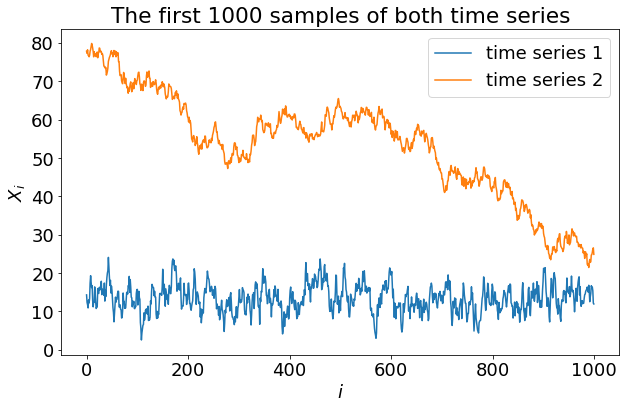## Introduction¶

In the first part of the error analysis tutorial we have introduced the binning analysis, an easy and common tool for error estimation. However, we have seen that it failed to deliver an estimate for our second data set. In this tutorial, we will get to know a different method: the autocorrelation analysis, sometimes also called auto covariance analysis. It not only delivers an estimate for the standard error of the mean (SEM), but also information on the correlations and the optimal sampling rate.

Before we start computing anything, we will give a brief overview over the relevant quantities and how they relate to each other. This outlines how one would go about computing these quantities. The end goal of this process is to define an estimator for the standard error of the mean $\sigma_\overline{X}$. And if the data allows for it, it can be calculated. If it fails, autocorrelation analysis provides more insight into the causes of the failure than the binning analysis from the first part of this tutorial. Albeit being more involved, it can provide a valuable tool for systems with difficult statistics.

Let us begin the theory by defining the auto-covariance function $R^{XX}(\tau)$ of an observable $X$, at lag time $\tau$:

\begin{align} R^{XX}(\tau) &\equiv \langle (X(t)-\langle X \rangle)(X(t+\tau)-\langle X \rangle) \rangle \\ &= \langle X(t) X(t+\tau) \rangle - \langle X \rangle^2, \tag{1} \end{align}

where $\langle \dots \rangle$ denotes the ensemble average of the expression inside the angled brackets — e.g. $\langle X \rangle$ is the true mean value of the observable $X$. In the previous part we have established an understanding of correlations as being the "similarity" of successive samples. This is an intuitive but inaccurate understanding. The auto-covariance function provides a means to measure and quantify correlation.

Computing the auto-covariance for $\tau=0$ yields the variance $\sigma=\langle X^2 \rangle - \langle X \rangle^2$. Normalizing the auto-covariance function by the variance yields the autocorrelation function (ACF)

\begin{align} A^{XX}(\tau) = \frac{R^{XX}(\tau)}{R^{XX}(0)} = \frac{\langle X(t) X(t+\tau) \rangle - \langle X \rangle^2}{\langle X^2 \rangle - \langle X \rangle^2}. \tag{2} \end{align}

The ACF can be used to estimate the correlation time $\tau_X$. Often, this can be simply done by fitting an exponential function to $A^{XX}$, from which we extract $\tau_{X, \mathrm{exp}}$ as the inverse decay rate. However, the ACF doesn't necessarily assume the shape of an exponential. That is when another quantity, called the integrated autocorrelation time

\begin{align} \tau_{X, \mathrm{int}} \equiv \int_0^\infty A^{XX}(\tau) \mathrm{d}\tau \tag{3} \end{align}

comes into play. Those two correlation times $\tau_{X, \mathrm{int}}$ and $\tau_{X, \mathrm{exp}}$ are identical for exponential ACFs, but if the ACF isn't exponential, $\tau_{X, \mathrm{int}}$ is the only meaningful quantity. It is related to the effective number of samples

\begin{align} N_\mathrm{eff} = \frac{N}{2 \tau_{X, \mathrm{int}}} \tag{4} \end{align}

and also to the standard error of the mean (SEM)

\begin{align} \sigma_\overline{X} = \sqrt{\frac{2 \sigma_X^2 \tau_{X, \mathrm{int}}}{N}} = \sqrt{\frac{\sigma_X^2}{N_\mathrm{eff}}}. \tag{5} \end{align}

where $\sigma_X^2 = \langle X^2 \rangle-\langle X \rangle ^2$ is the variance of the observable $X$.

## Computing the auto-covariance function¶

Equations (1) and (2) involve an infinite, continuous time series $X(t)$. In the simulation world however, we work with finite, discrete time series. These limitations dictate how we can estimate the true (unknown) autocorrelation function. For a finite, time-discrete set of samples $X_i$, a commonly used estimator is the following expression

\begin{align} \hat{R}^{XX}_j = \frac{1}{N} \sum^{N-|j|}_{i=1}(X_i-\overline{X})(X_{i+|j|}-\overline{X}), \tag{6} \end{align}

where $N$ is the total number of samples, and $\overline{X}=\frac{1}{N}\sum_{i=1}^N X_i$ is the average of all samples. This estimates the auto-covariance function at lag time $\tau=j\Delta t$ where $\Delta t$ is the time separation between samples.

Before we continue, we want to notify the reader about a few subtleties regarding this estimator:

• Ideally, we would use $\langle X \rangle$ instead of $\overline{X}$, since the latter is only an estimate of the former. In most cases we don't know $\langle X \rangle$, thus we introduce a small unknown bias by using the estimated mean $\overline{X}$ instead.
• Actually, the sum does not contain $N$ terms, but $N-|j|$ terms. Consequently, we should divide the whole sum by $N-|j|$ and not by $N$. In fact, this approach yields a different estimator to the auto-covariance function (the so-called unbiased estimator). However, for large $N$ and small $j$, both estimators yield similar results. This is why the simpler $N$ is commonly used anyway.

#### Exercise¶

Compute the auto-covariance function of the data in time_series_1 using the estimator in equation (6) and store it into a numpy array called autocov. Compute it for all $j$ from 0 up to 999. Plot it against $j$.

In :
# naive Python solution
autocov = np.zeros(300)
avg = np.average(time_series_1)
for j in range(300):
temp = 0.
for i in range(N_SAMPLES - j):
temp += (time_series_1[i] - avg) * (time_series_1[i + j] - avg)
autocov[j] = temp / N_SAMPLES

fig = plt.figure(figsize=(10, 6))
plt.plot(autocov)
plt.xlabel("lag time $j$")
plt.ylabel("$\hat{R}^{XX}_j$")
plt.show()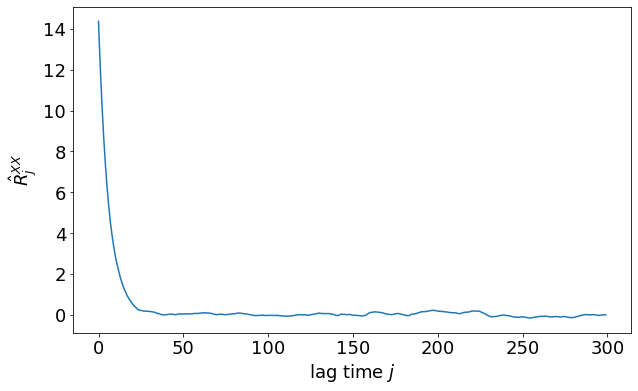Depending on your implementation, this computation might have taken a significant amount of time (up to a couple tens of seconds). When doing a lot of these computations, using highly optimized routines for numerics can save a lot of time. The following example shows how to utilize the common Numpy package to do the job quicker.

In :
# Numpy solution
time_series_1_centered = time_series_1 - np.average(time_series_1)
autocov = np.empty(1000)

for j in range(1000):
autocov[j] = np.dot(time_series_1_centered[:N_SAMPLES - j], time_series_1_centered[j:])
autocov /= N_SAMPLES

fig = plt.figure(figsize=(10, 6))
plt.gca().axhline(0, color="gray", linewidth=1)
plt.plot(autocov)
plt.xlabel("lag time $j$")
plt.ylabel("$\hat{R}^{XX}_j$")
plt.show()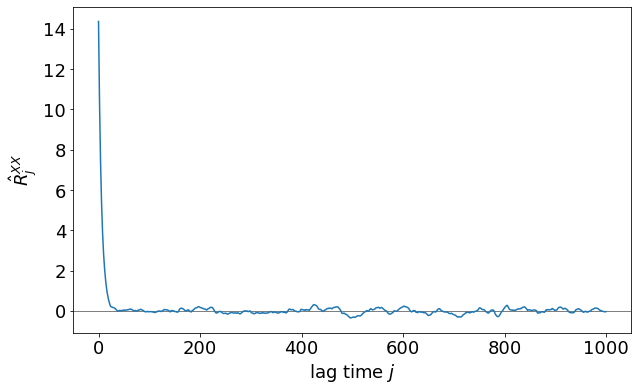We can see that the auto-covariance function starts at a high value and decreases quickly into a long noisy tail which fluctuates around zero. The high values at short lag times indicate that there are strong correlations at short time scales, as expected. However, even though the tail looks uninteresting, it can bear important information about the statistics of your data. Small systematic deviations from 0 in the tail can be a hint that long-term correlations exist in your system. On the other hand, if there is no sign of a systematic deviation from 0 in the tail, this usually means that the correlation is decaying well within the simulation time, and that the statistics are good enough to estimate an error. In the above example, the correlation quickly decays to zero. Despite the noise in the tail, the statistics seem very reasonable.

## Autocorrelation time¶

Continuing our example, we can zoom into the first part of the auto-covariance function (using a log scale). We see that it indeed does have similarities with an exponential decay curve. In general, it isn't an exponential, but often can be approximated using one. If it matches reasonably well, the inverted prefactor in the exponential can be directly used as the correlation time, which is a measure for how many sampling intervals it takes for correlations to decay. Execute the following code cell for an illustration.

In :
from scipy.optimize import curve_fit

def exp_fnc(x, a, b):
return a * np.exp(-x / b)

N_MAX = 1000
j = np.arange(1, N_MAX)
j_log = np.logspace(0, 3, 100)
popt, pcov = curve_fit(exp_fnc, j, autocov[1:N_MAX], p0=[15, 10])

# compute analytical ACF of AR(1) process
AN_SIGMA_1 = np.sqrt(EPS_1 ** 2 / (1 - PHI_1 ** 2))
AN_TAU_EXP_1 = -1 / np.log(PHI_1)
an_acf_1 = AN_SIGMA_1**2 * np.exp(-j / AN_TAU_EXP_1)

fig = plt.figure(figsize=(10, 6))
plt.plot(j, autocov[1:N_MAX], "x", label="numerical ACF")
plt.plot(j, an_acf_1, "-.", linewidth=3, label="analytical ACF")
plt.plot(j_log, exp_fnc(j_log, popt, popt), label="exponential fit")
plt.xlim((1, N_MAX))
plt.xscale("log")
plt.xlabel("lag time $j$")
plt.ylabel("$\hat{R}^{XX}_j$")
plt.legend()
plt.show()

print(f"Exponential autocorrelation time: {popt:.2f} sampling intervals")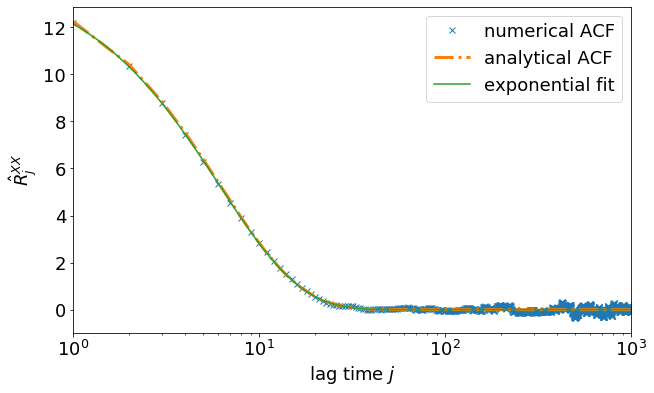Exponential autocorrelation time: 6.15 sampling intervals


Since the auto-covariance function is very well matched with an exponential, this analysis already gives us a reasonable estimate of the autocorrelation time. Here we have the luxury to have an analytical ACF at hand which describes the statistics of the simple AR(1) process, which generated our simulation data. It is in fact exponential and agrees very well with the numerical ACF. In practice, however, you will neither know an analytical ACF, nor know if the ACF is exponential, at all. In many systems, the ACF is more or less exponential, but this is not necessarily the case.

For the sake of completeness, we also want to compute the integrated correlation time. This technique must be applied when the ACF is not exponential. For that purpose, we first need to normalize the auto-covariance function in order to get the autocorrelation function (as opposed to auto-covariance function), and then integrate over it.

The integration in equation (3) is again approximated as a discrete sum over the first $j_\mathrm{max}$ values of the ACF (except $\hat{A}^{XX}_0$, which is always 1):

\begin{align} \hat{\tau}_{X, \mathrm{int}} = \frac{1}{2} + \sum_{j=1}^{j_\mathrm{max}} \hat{A}^{XX}_j \tag{7} \end{align}

where $\hat{A}^{XX}_j = \hat{R}^{XX}_j / \hat{R}^{XX}_0$ is the estimated ACF. The sum is evaluated up to a maximum number of terms $j_\mathrm{max}$. This maximum number of terms is a crucial parameter. In the following code cell, $\hat{\tau}_{X, \mathrm{int}}$ is plotted over $j_\mathrm{max}$.

In :
# compute the ACF
acf = autocov / autocov

# integrate the ACF (suffix _v for vectors)
j_max_v = np.arange(1000)
tau_int_v = np.zeros(1000)
for j_max in j_max_v:
tau_int_v[j_max] = 0.5 + np.sum(acf[1:j_max + 1])

# plot
fig = plt.figure(figsize=(10, 6))
plt.plot(j_max_v[1:], tau_int_v[1:], label="numerical summing")
plt.plot(j_max_v[(1, -1),], np.repeat(AN_TAU_EXP_1, 2), "-.", label="analytical")
plt.xscale("log")
plt.xlabel(r"sum length $j_\mathrm{max}$")
plt.ylabel(r"$\hat{\tau}_{X, \mathrm{int}}$")
plt.legend()
plt.show()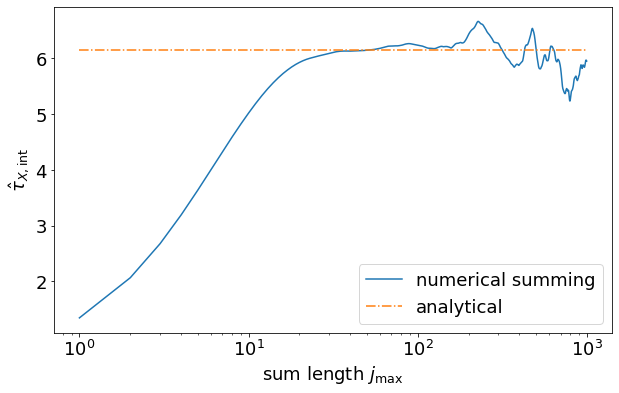In this plot, we have the analytical solution at hand, which is a luxury not present in real applications. For the analysis, we therefore need to act as if there was no analytic solution:

We see that the integrated autocorrelation time seems to quickly reach a plateau at a $j_\mathrm{max}$ of around 20. Further summation over the noisy tail of the ACF results in a random-walky behaviour. And for even larger $j_\mathrm{max}$, the small unknown bias of the ACF starts to accumulate, which is clearly unwanted. Thus, we have to find a good point to cut off the sum. There are several ways to determine a reasonable value for $j_\mathrm{max}$. Here, we demonstrate the one by A. Sokal , who states that it performs well if there are at least 1000 samples in the time series. We take the smallest $j_\mathrm{max}$, for which the following inequality holds:

$j_\mathrm{max} \geq C \times \hat{\tau}_{X, \mathrm{int}}(j_\mathrm{max}) \tag{8}$

where $C$ is a constant of about 5, or higher if convergence of $\hat{\tau}_{X, \mathrm{int}}$ is slower than an exponential (up to $C=10$). In the following code cell, we plot the left side against the right side, and determine $j_\mathrm{max}$.

In :
C = 5.0

# determine j_max
j_max = 0
while j_max < C * tau_int_v[j_max]:
j_max += 1

# plot
fig = plt.figure(figsize=(10, 6))
plt.plot(j_max_v[1:], C * tau_int_v[1:])
plt.plot(j_max_v[1:], j_max_v[1:])
plt.plot([j_max], [C * tau_int_v[j_max]], "ro")
plt.xscale("log")
plt.ylim((0, 50))
plt.xlabel(r"sum length $j_\mathrm{max}$")
plt.ylabel(r"$C \times \hat{\tau}_{X, \mathrm{int}}$")
plt.show()

print(f"j_max = {j_max}")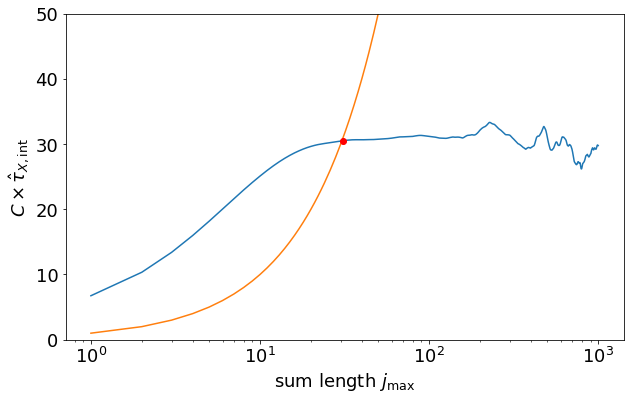j_max = 31


Using this value of $j_\mathrm{max}$, we can calculate the integrated autocorrelation time $\hat{\tau}_{X, \mathrm{int}}$ and estimate the SEM with equation (5).

In :
tau_int = tau_int_v[j_max]
print(f"Integrated autocorrelation time: {tau_int:.2f} time steps\n")

N_eff = N_SAMPLES / (2 * tau_int)
print(f"Original number of samples: {N_SAMPLES}")
print(f"Effective number of samples: {N_eff:.1f}")
print(f"Ratio: {N_eff / N_SAMPLES:.3f}\n")

sem = np.sqrt(autocov / N_eff)
print(f"Standard error of the mean: {sem:.4f}")

Integrated autocorrelation time: 6.10 time steps

Original number of samples: 100000
Effective number of samples: 8196.2
Ratio: 0.082

Standard error of the mean: 0.0419


#### Exercise¶

• Write a function called autocorrelation_analysis, which takes as arguments

• data (a numpy array containing the time series to be analyzed),
• C (which is the criterion to find $j_\mathrm{max}$) and
• window (an integer that defines how much of the auto-covariance function is computed during the analysis).

The function shall return the SEM and logging.info out:

• mean
• SEM
• integrated autocorrelation time
• effective number of samples.

It should also make a plot of the autocorrelation function and the integrated ACF. You can adapt the other examples and solutions in this notebook for this function.

• Use this function to analyze time_series_2.

This function can serve as a template for the analysis of your own simulation data.

In :
def autocorrelation_analysis(data, C, window):
# initial processing
data_size = len(data)
avg = np.average(data)
data_centered = data - avg

# auto-covariance function
autocov = np.empty(window)
for j in range(window):
autocov[j] = np.dot(data_centered[:data_size - j], data_centered[j:])
autocov /= data_size

# autocorrelation function
acf = autocov / autocov

# integrate autocorrelation function
j_max_v = np.arange(window)
tau_int_v = np.zeros(window)
for j_max in j_max_v:
tau_int_v[j_max] = 0.5 + np.sum(acf[1:j_max + 1])

# find j_max
j_max = 0
while j_max < C * tau_int_v[j_max]:
j_max += 1

# wrap it up
tau_int = tau_int_v[j_max]
N_eff = data_size / (2 * tau_int)
sem = np.sqrt(autocov / N_eff)

# create ACF plot
fig = plt.figure(figsize=(10, 6))
plt.gca().axhline(0, color="gray",linewidth=1)
plt.plot(acf)
plt.xlabel("lag time $j$")
plt.ylabel("$\hat{R}^{XX}_j$")
plt.show()

# create integrated ACF plot
fig = plt.figure(figsize=(10, 6))
plt.plot(j_max_v[1:], C * tau_int_v[1:])
plt.ylim(plt.gca().get_ylim()) # explicitly keep the limits of the first plot
plt.plot(j_max_v[1:], j_max_v[1:])
plt.plot([j_max], [C * tau_int_v[j_max]], "ro")
plt.xscale("log")
plt.xlabel(r"sum length $j_\mathrm{max}$")
plt.ylabel(r"$C \times \hat{\tau}_{X, \mathrm{int}}$")
plt.title("")
plt.show()

# print out stuff
print(f"Mean value: {avg:.4f}")
print(f"Standard error of the mean: {sem:.4f}")
print(f"Integrated autocorrelation time: {tau_int:.2f} time steps")
print(f"Effective number of samples: {N_eff:.1f}")

return sem

sem_2 = autocorrelation_analysis(time_series_2, 5, 20000)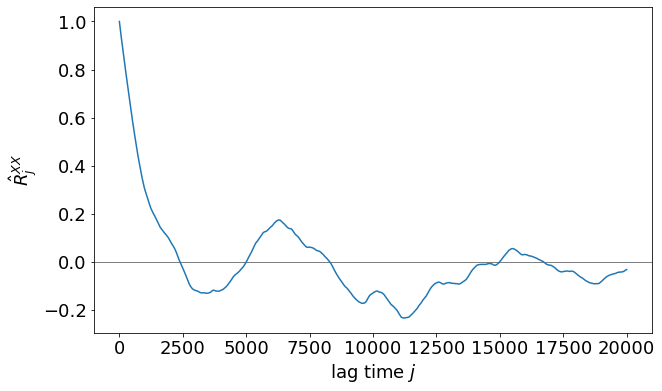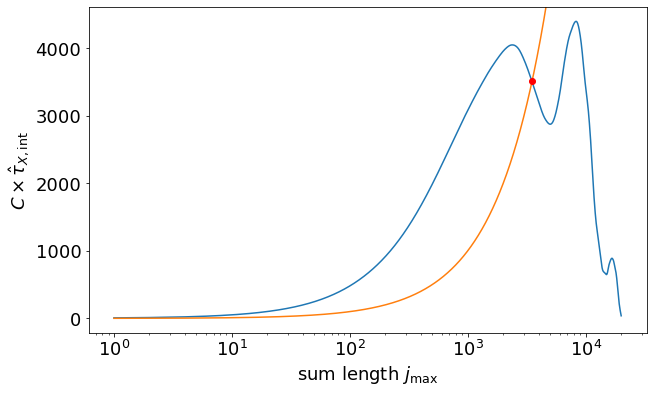Mean value: 43.1782
Standard error of the mean: 2.7456
Integrated autocorrelation time: 701.00 time steps
Effective number of samples: 71.3


#### Exercise¶

Interpret the results of the analysis of time_series_2.

Interpretation of the analysis

Even though the autocorrelation analysis spits out a number for the SEM, it cannot be trusted. The ACF has a lot of noise in its tail which lets the integrated ACF become very "random walky" and therefore unreliable. This means that the ACF has not properly decayed to zero. The only possibility to get better statistics is to simulate for a longer time. Since the autocorrelation time is very long, it is sufficient to store a lot less samples during simulation. The sampling interval could be chosen to be 100 times larger and still capture the statistics sufficiently well.